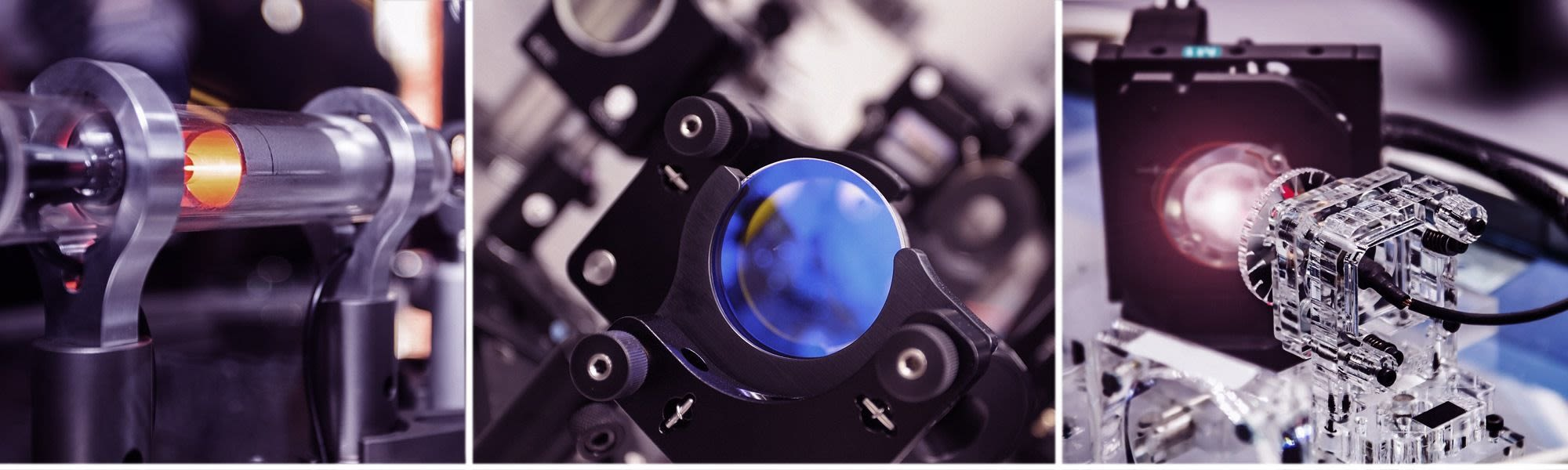My shopping cartSupport & Info
• ››
• ››
• ››
• ››
• ››
• ››
• ››
• ››
• ››
• ››
• ››
• ››
• ››
• ››
• ››
• Optical Handbook ch 1

Chapter 1 - General Rules

In the application of optical systems we may need to apply some simple rules. Often it is not necessary to know exactly the quantity or the structure wanted: an order of magnitude or a “rule of thumb” is enough. This manual provides some of these useful rules. Many other information may be found in “Photonics Rules of Thumb” (1996) by John Lester Miller and Ed Friedman, McGraw-Hill Co. Inc..

OPTICS *

- In the optic systems light always travels from left to right. If the surface of an optic system is convex (with respect to the direction of light) then it has a positive radius of curvature; while if it is concaveit has a negative radius of curvature.Fig. 1-Sign of curved surfaces

- The focal length of a lens or of an objective always holds to paraxial rays: indeed it does not take into account of aberrations effects resulting for non paraxial rays. When the distance between the last surface of an optical system (from the center of the surface) and the focus is measuredby means of parallel light beam, it means that its “back-focus” is measured. The focal length is measured from the principal plane and not from the last surface. However, for this measurement, a small dimensions diaphragm, with respect to the lens or objectivediameter, must be used. The“flange distance” is the distance between a mechanical reference placed on an objective and the focal plane. Many standard distances are measured from this plane. For example the mount “C” standard distance is17.526 mm (0.690 inches).

- Object-lens and lens-image distance . The distances are always measured from the lens (or objective)“principal plane”. The principal planeofa simplelens is located in the center of the lens or close to one of its surfaces. In a complex objective it may be distant.Fig. 2 - Object-image relation- The lens verse: the surface with the largest radius of curvature must always be faced by the most distant conjugate.

- The reflectivity of a metal can be roughly estimated by the formula:where R is the percentage reflectivity, ρ is the resistivity of the metal expressed in micro-ohm per meter and λ is the wavelength in microns.

- If, giving the specifications of a coating, the polarization can be s or p, then is preferable to reflect s and transmit p.

If a coating (i.e. anti-reflective or other treatment of the surface of the optical system) has been optimized for normal incidence, when you rotate it of 45 degree, the wavelength for which the coating is optimized decreases ofabout 10%.

- The "stray light" of the holographic gratings is usually better than an order of magnitude compared to the equivalent obtained by mechanical ruling.

- The focal length of a plano-convex or plano-concave lens is about twice the radius of curvature of the convex or concave surface. The sign of the focal length is positive for a convex surface while it is negative for a concave surface.

- The focal length of a bi-concave or bi-convex lens with two equal radii of curvature, is equal to the radius of curvature (i.e. negative in the case of bi-concave lens, positive in the case of bi-convex lens).

- The insertion in air of a waveplate in the space preceding or following an optical system increases the optical path in that space of approximately 1/3 of the waveplate thickness.

- The deviation δ produced by a prism with a not too large vertex angle α is roughly given by:where n' is the refraction index of the prism material.

- In almost all applications where spherical aberration is present (present along the axis only) the best image is obtained when the lens is focused at the point of minimum spot size.

- The detector responsivity (in amp / watt) is approximately equal to its quantum efficiency divided by 1.24 •wavelength expressed in microns.

- The coherence length (in meters) of a light source is determined by the formula:where c is the speed of light in meters per second (3 • 10 m/s) and ∆υ is the width of the source spectral line expressed in Hertz.

If the width of the spectral line of a source is given in nanometers (1 nm = 10 Angstrom), then to convert the line width in Hertz, you just divide it by 3 • 10.

- The spot diameter of a focused laser beam can be evaluated with the formula:where λ is the wavelength oflaser, M the beam quality and E is the divergence.

- The approximated resolving power (expressed in lines per mm) of an optical fibers bundle is 500 divided by thediameter in microns of the bundleitself.

- The equation:provides an approximate relationship between the core diameter D, the numerical aperture NA (see page 45 et seq.) and the cutoff wavelength λc in a single mode optical fiber.

CCD SIZEFig. 3 - CCD SIZE (mm)